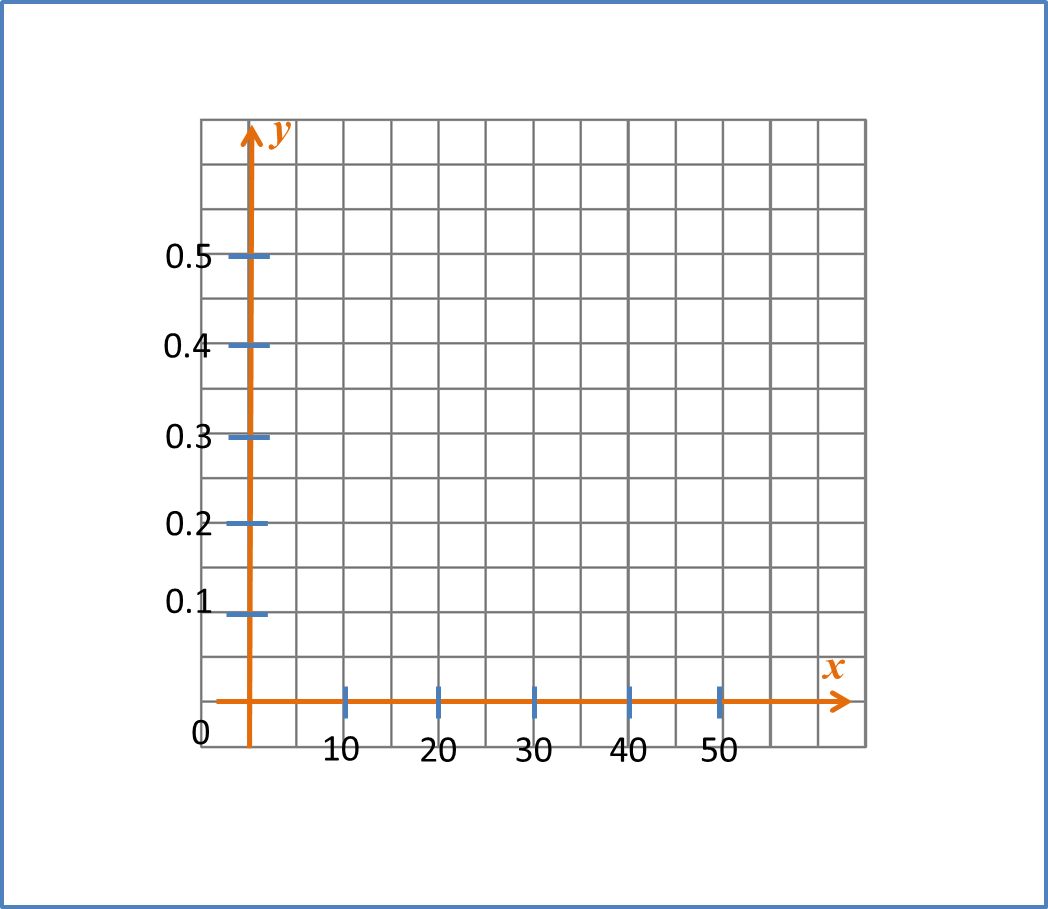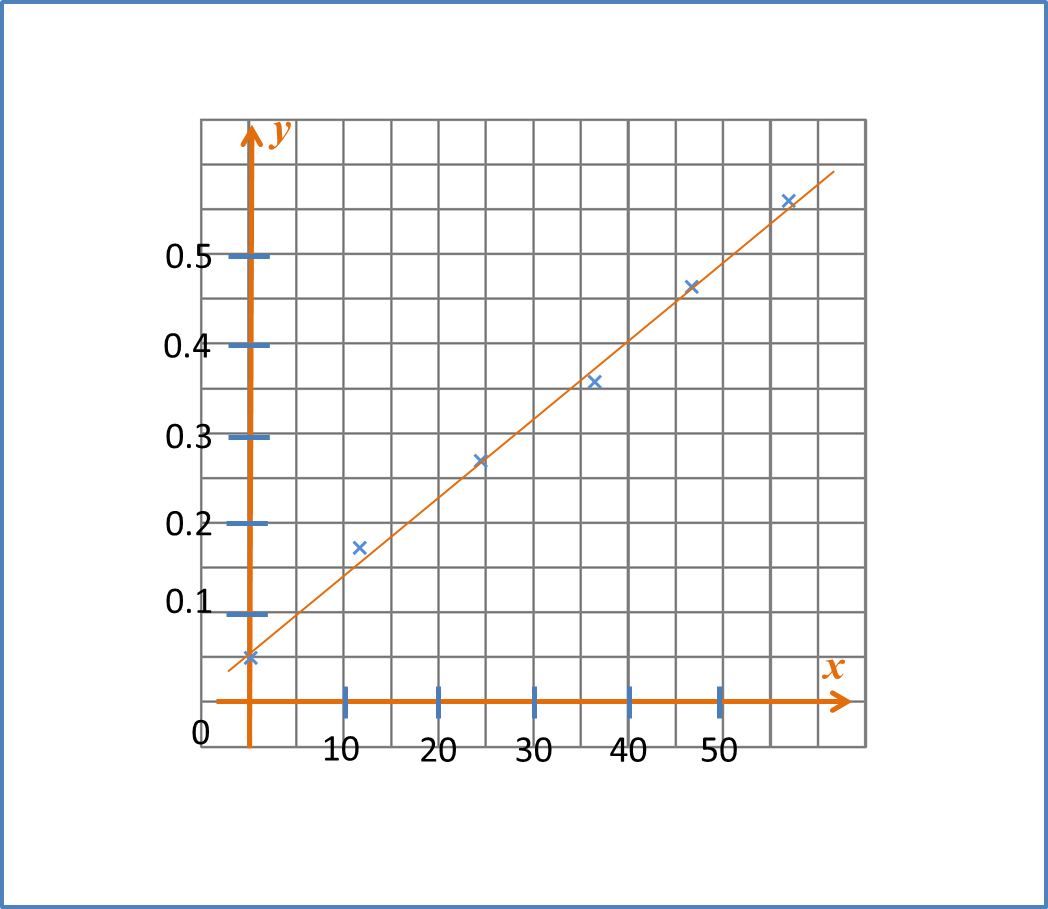# 2.2.1 Steps to draw the Line of Best Fit

Steps to draw a line of Best Fit
(i) Select suitable scales for the x-axis and the y-axis, make sure the points plotted accurately and the graph produced is large enough on the graph paper,
(ii) Mark the points correctly,
(iii) Use a long and transparent ruler to draw the line of best fit.

Step 1 : Select the suitable scale on x and y axis

(the graph produced must be more than 50% of the graph paper)Step 2 : Mark the points correctlyStep 3 : Draw the Line of Best Fit

* Note
-the line passes through four points
-one point is above the line
-one point is below the line